#Symmetry of Alphabets, Numbers & Shapes

This page will helps you to understand the concepts of symmetry, lines of symmetry, types of symmetry along with example alphabets A to Z ,numbers 0 to 9 and major geometry shapes.

Symmetry : The dotted line divides the figure into two identical parts. When the figure is folded about this line the two parts fir exactly over each other. Such a figure is said to be Symmetrical.

Axis of Symmetry : THe line that divides the figure into two identical parts is called Axis of Symmetry.

Vertical Line of Symmetry :The axis of the shape which divides the shape into two identical halves, vertically, is called a vertical line of symmetry.

Horizontal Line of Symmetry :The symmetry line or horizontal axis of a shape which divides the shape into two identical halves is known as horizontal line of symmetry

1) How Many line / lines of symmetry the given figure have ?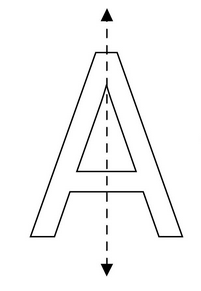Answer: The given figure have 1 line of symmetry.

Types of lines of symmetry is Vertical Symmetry.

2) How Many line / lines of symmetry the given figure have ?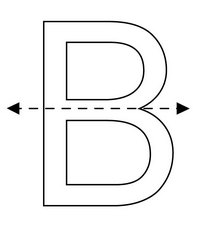Answer: The given figure have 1 line of symmetry.

Types of lines of symmetry is Horizontal Symmetry.

3) How Many line / lines of symmetry the given figure have ?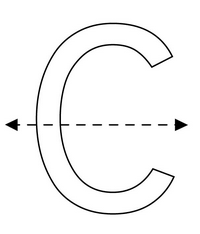Answer: The given figure have 1 line of symmetry.

Types of lines of symmetry is Horizontal Symmetry.

4) How Many line / lines of symmetry the given figure have ?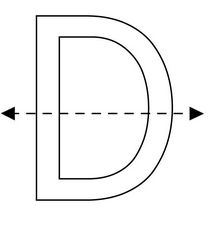Answer: The given figure have 1 line of symmetry.

Types of lines of symmetry is Horizontal Symmetry.

5) How Many line / lines of symmetry the given figure have ?Answer: The given figure have 1 line of symmetry.

Types of lines of symmetry is Horizontal Symmetry.

6) How Many line / lines of symmetry the given figure have ?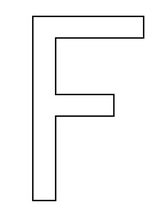Answer: The given figure have zero line of symmetry.

7) How Many line / lines of symmetry the given figure have ?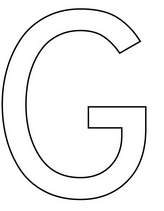Answer: The given figure have zero line of symmetry.

8) How Many line / lines of symmetry the given figure have ?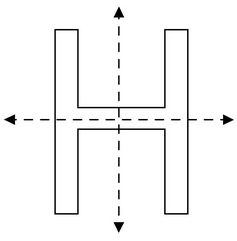Answer: The given figure have 2 lines of symmetry.

Types of lines of symmetry is Vertical and Horizontal Symmetry.

9) How Many line / lines of symmetry the given figure have ?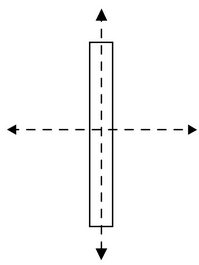Answer: The given figure have 2 lines of symmetry.

Types of lines of symmetry is Vertical and Horizontal Symmetry.

10) How Many line / lines of symmetry the given figure have ?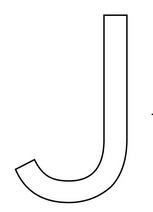Answer: The given figure have zero line of symmetry.

11) How Many line / lines of symmetry the given figure have ?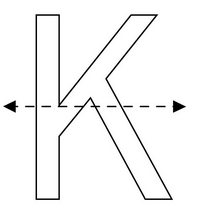Answer: The given figure have 1 line of symmetry.

Types of lines of symmetry is Horizontal Symmetry.

12) How Many line / lines of symmetry the given figure have ?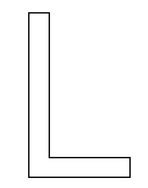Answer: The given figure have zero line of symmetry.

13) How Many line / lines of symmetry the given figure have ?Answer: The given figure have 1 line of symmetry.

Types of lines of symmetry is Vertical Symmetry.

14) How Many line / lines of symmetry the given figure have ?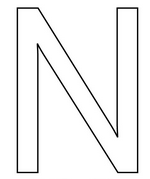Answer: The given figure have zero line of symmetry.

15) How Many line / lines of symmetry the given figure have ?Answer: The given figure have 2 lines of symmetry.

Types of lines of symmetry is Vertical and Horizontal Symmetry.

16) How Many line / lines of symmetry the given figure have ?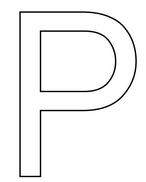Answer: The given figure have zero line of symmetry.

17) How Many line / lines of symmetry the given figure have ?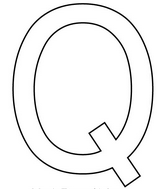Answer: The given figure have zero line of symmetry.

18) How Many line / lines of symmetry the given figure have ?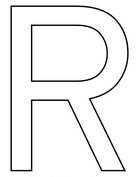Answer: The given figure have zero line of symmetry.

19) How Many line / lines of symmetry the given figure have ?Answer: The given figure have zero line of symmetry.

20) How Many line / lines of symmetry the given figure have ?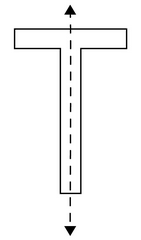Answer: The given figure have 1 line of symmetry.

Types of lines of symmetry is Vertical Symmetry.

21) How Many line / lines of symmetry the given figure have ?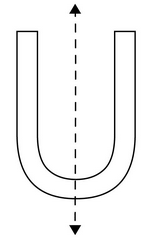Answer: The given figure have 1 line of symmetry.

Types of lines of symmetry is Vertical Symmetry.

22) How Many line / lines of symmetry the given figure have ?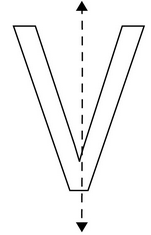Answer: The given figure have 1 line of symmetry.

Types of lines of symmetry is Vertical Symmetry.

23) How Many line / lines of symmetry the given figure have ?Answer: The given figure have 1 line of symmetry.

Types of lines of symmetry is Vertical Symmetry.

24) How Many line / lines of symmetry the given figure have ?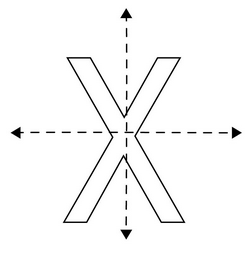Answer: The given figure have 2 lines of symmetry.

Types of lines of symmetry is Vertical and Horizontal Symmetry.

25) How Many line / lines of symmetry the given figure have ?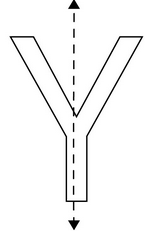Answer: The given figure have 1 line of symmetry.

Types of lines of symmetry is Vertical Symmetry.

26) How Many line / lines of symmetry the given figure have ?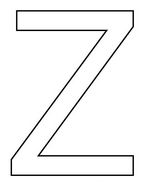Answer: The given figure have zero line of symmetry.

Types of Lines of Symmetry :

Letters with vertical symmetry are: A, H, I, M, O, T, U, V, W, X, Y

Letters with horizontal symmetry are: B, C, D, E, H, I, K, O, X

Letters with both vertical and horizontal symmetry are: H, I, O, X

Lines of Symmetry :

Letters with 0 line of symmetry are: F, G, J, L, N, P, Q, R, S, Z

Letters with 1 line of symmetry are: A, B, C, D, E, K, M, T, U, V, W, Y

Letters with 2 lines of symmetry are: H, I, O, X

1) How Many line / lines of symmetry in Numbers From 0 To 9 have ?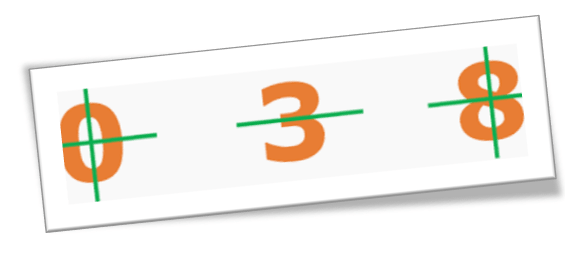Number 0 has 2 lines of symmetry. (1 Horizontal and 1 Vertical)

Number 1 and 2 has no symmetry.

Number 3 has one horizontal line of symmetry.

Number 4, 5, 6 and 7 has no symmetry.

Number 8 has 2 lines of symmetry. (1 Horizontal and 1 Vertical)

Number 9 has no symmetry.

1) How Many line / lines of symmetry in major geometry shapes have ?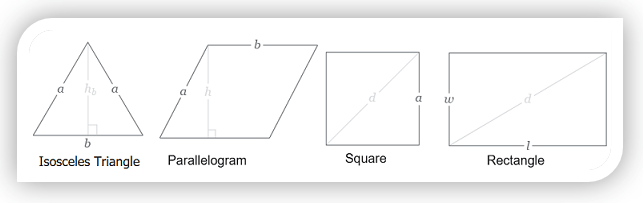Isosceles Triangle has 1 line of symmetry.

Parallelogram has zero line of symmetry.

Square has 4 lines of symmetry.

Rectangle has 2 lines of symmetry.###Recommended Pages ►

Online Algebra calculation, formulas

Online math number calculation, formulas

Statistical calculation

Matrix calculation, formulas

Other calculators